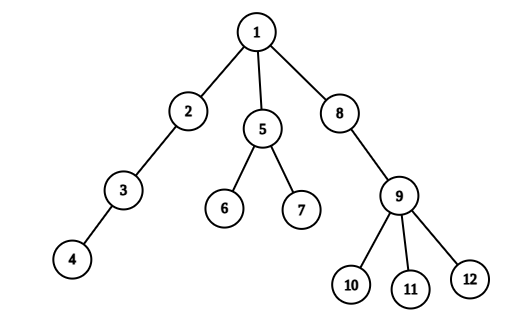0

# Ninja Land

Difficulty: NINJAContributed By
Arindam Majumder
Avg. time to solve
100 min
Success Rate
20%

Problem Statement

#### For Example:

``````Suppose Ninja is currently standing at X city and want to visit Y city. Let the heights of all the cities in the path from X to Y(including X and Y) are 10 20 5 30. Now these series of heights forms and alternate series. So Ninja will visit the city Y.

Some examples of alternate series are 15 4 11 8 25, 100 120 50 70 60 but the series like 3 5 4 1, 6 3 10 12 are not alternating.
``````
##### Now you will be asked q queries, and there are two types of queries:
``````1 X Y: change the height of city X to Y.

2 X Y: Check whether the path between city X to Y is alternating or not.
``````
##### Input Format:
``````The first line contains two space-separated integers ‘N’ and ‘Q’ denoting the number of cities in the Ninja Land and the number of queries.

The next N-1 lines will contain two space-separated integers X and Y, denoting that there is an undirected edge between city X and city Y.

The next line contains ‘N’ space-separated integers denoting the initial heights of each city.

The next q lines contain three space-separated integers representing the queries of both types.
``````
##### Output Format:
``````Output for each query of type two only, whether the path between the two cities of that query is alternating or not.

Output for each query will be printed in a separate line.
``````
##### Note
``````You are not required to print anything; it has already been taken care of. Just implement the function.
``````
##### Constraints:
``````1 <= N,Q <= 10^5
1 <= X,Y <= N
1 <= height[i] <= 10^9

Where N is the number of cities, Q is the number of queries, X and Y represent the cities, and height[i] represents the height of the i’th city.

Time Limit: 1 sec.
``````
##### Sample Input 1:
``````12 3
1 2
2 3
3 4
1 5
5 6
5 7
1 8
8 9
9 10
9 11
9 12
10 8 5 9 12 16 8 18 21 11 19 20
2 4 7
1 1 6
2 4 7
``````
##### Sample Output 1:
``````NO
YES
``````
##### Explanation For Sample Output 1:``````In the first query, we have to check whether the path between city 4 and city 7 is alternating or not. The path between 4 and 7 is 4, 3, 2, 1, 5, 6, 7 and their corresponding height is 9, 5, 8, 10, 12, 8.
This sequence is not alternating as the 3rd 4th and 5th indices are strictly increasing. So, the output will be NO.

In the second query, we have to update the height array. The height of city 1 will be 6.
The final height array will be 6 8 5 9 12 16 8 18 21 11 19 20.

In the third query, we have to check whether the path between city 4 and city 7 is alternating or not. The path between 4 and 7 is 4, 3, 2, 1, 5, 6, 7 and their corresponding height is 9, 5, 8, 6, 12, 8.
Clearly, this is an alternating sequence. So, the output will be YES.
``````
##### Sample Input 2:
``````6 4
1 5
2 1
3 2
4 3
4 6
2 5 4 1 6 3
2 3 3
1 2 7
2 5 6
2 5 1
``````
##### Sample Output 2:
``````YES
NO
YES
``````Console